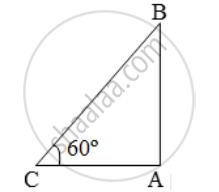# The shadow of a building is 20 m long when the angle of elevation of the sun is 60º. Find the height of the building - Mathematics

Sum

The shadow of a building is 20 m long when the angle of elevation of the sun is 60º. Find the height of the building

#### Solution

Let AB be the building and AC be its shadow.Then, AC = 20 m and ∠ACB = 60º.

Let AB = h.

Then,

\frac { AB }{ AC } = tan 60º = √3

⇒ h/20 = √3

∴ h = (20 × √3 )m = (20 × 1.732) m

= 34.64 m.

Concept: Heights and Distances
Is there an error in this question or solution?